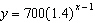Name:    MCR3U - Unit 3 Practice Test

Multiple Choice
Identify the choice that best completes the statement or answers the question.

1.

A colony of ants has an initial population of 200 and doubles every day. A function that can be used to model the ant population, p, after t days is
 a.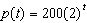c.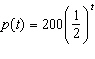b.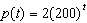d.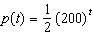2.

An investment of \$100 is placed into an account that earns interest, compounded annually, at a rate of 5% for 7 years. The amount, A, in the account can be modelled by the function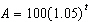, where t is the time, in years. What is the range of this function?
 a.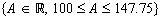c.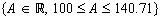b.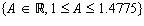d.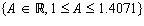3.

The expression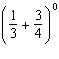is equal to
 a.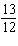c.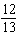b. 1 d. 0

4.

Simplify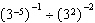.
 a.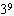c.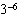b. 3 d.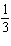5.

Simplify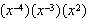. Express your answer using only positive exponents.
 a.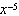c.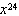b.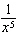d.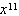6.

Simplify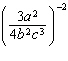. Express your answer using only positive exponents.
 a.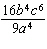c.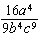b.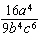d.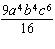7.

The equation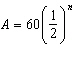can also be written as
 a.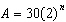c.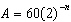b.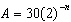d.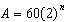8.

Evaluate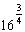.
 a. 8 c. 40.3 b. 196 608 d.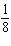9.

Which of the following expressions is equivalent to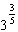?
 a.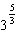c.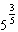b. 3 d.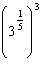10.

When simplified and written with positive exponents, the expression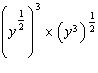simplifies to
 a.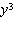c.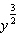b. y d.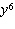11.

Which exponential equation matches the graph shown?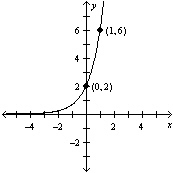a.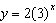c.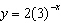b.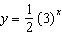d.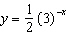12.

Which exponential equation matches the graph shown?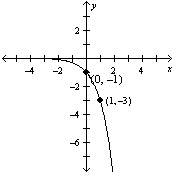a.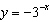c.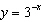b.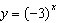d.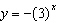13.

Which of the following transformations maps the function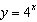onto the function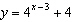?
 a. a horizontal shift 3 units to the right and a vertical shift 4 units up b. a horizontal shift 3 units to the left and a vertical shift 4 units up c. a horizontal shift 3 units to the right and a vertical shift 4 units down d. a horizontal shift 3 units to the left and a vertical shift 4 units down

14.

Which function results when the graph of the function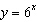is translated 2 units to the right and 1 unit up?
 a.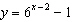c.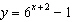b.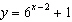d.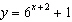15.

For the exponential function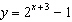, which properties does the graph of the function have?
 a. domain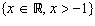, range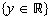, asymptote at y = –1 c. domain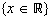, range, asymptote at y = –1 b. domain, range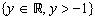, asymptote at y = –1 d. domain, range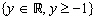, asymptote at y = 1

16.

Which equation can be used to model the given information?
 Year (x) Population (y) 0 700 1 728 2 757 3 787 4 820 5 854
 a.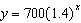c.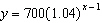b.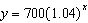d.